Ch 2. Particle Force and Acceleration Multimedia Engineering Dynamics Rect.Coord. Normal/Tang. Coord. PolarCoord. Orbital Mechanics Computational Mechanics
 Chapter - Particle - 1. General Motion 2. Force & Accel. 3. Energy 4. Momentum - Rigid Body - 5. General Motion 6. Force & Accel. 7. Energy 8. Momentum 9. 3-D Motion 10. Vibrations Appendix Basic Math Units Basic Equations Sections Search eBooks Dynamics Fluids Math Mechanics Statics Thermodynamics Author(s): Kurt Gramoll ©Kurt GramollDYNAMICS - CASE STUDY SOLUTION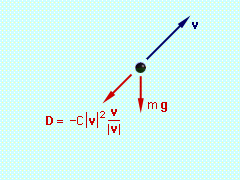Force Diagram - Vectors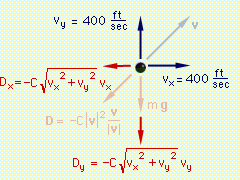Force Diagram - Components Begin with a free-body diagram. The velocity has been included on the free-body diagram for reference. To apply Eqs. 5, the x and y components of the total force on the projectile must be determined. Since v/|v| is a unit vector, D can be written as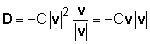The external forces are weight and drag,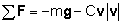The x and y components of the total force areNow, consider the case when C = 0.002, and let Δt = 0.1. At the initial time to      to = 0      vx(to) = 400 ft/s           vy(to) = 400 ft/s Using the four basic equations for position and velocity (Eqs. 5) the following is determined, x - position      x(to + Δt) = x(to) + vx(to) Δt      x(0 + 0.1) = 0 + 400 (0.1)                     = 40 ft y - position      y(to + Δt) = y(to) + vy(to) Δt      y(0 + 0.1) = 0 + 400(0.1)                     = 40 ft x - velocity                                   vx(to + Δt) = vx(to) + (1/m) ΣFx(to, x(to), y(to), vx(to), vy(to))Δt                                   vx(0 + 0.1) = vx(0) - (C/m) vx(0) (vx 02 + vy 02)0.5 Δt                                   vx(0.1) = 400 - (0.002/100) (400) (4002 + 4002)0.5 (0.1)                                              = 399.6 ft/s                                x - velocity                                    vy(to + Δt) = vy(to) + (1/m) ΣFy(to, x(to), y(to), vx(to), vy(to))Δt                                    vy(0 + 0.1) = vy(0) - g Δt - (C/m) vx (0) (vx 02 + vy 02)0.5 Δt                                    vy(0 + 0.1) =  400 - 32.2(0.1) - (0.002/100) (400) (4002 + 4002)0.5 (0.1)                                                      = 396.3 ft/s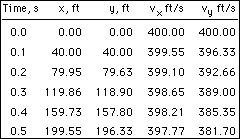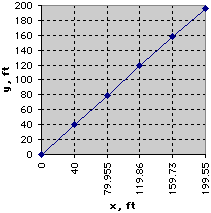Results Plot Continuing this way, the results for the first five time-steps can be obtained as shown in the table at the left. These values can then be plotted to obtain an approximate solution for the trajectory of the projectile: When there is no drag (C = 0), the closed-form solution for the trajectory can be obtained and compared with the numerical solution. The graph below presents this comparison using Δt = 3.5s, Δt = 1.0s, and Δt = 0.1s. Notice that the numerical solution with Δt = 0.1s is the closest to the exact solution. This indicates that as the time-step is decreased, the numerical solution becomes more accurate.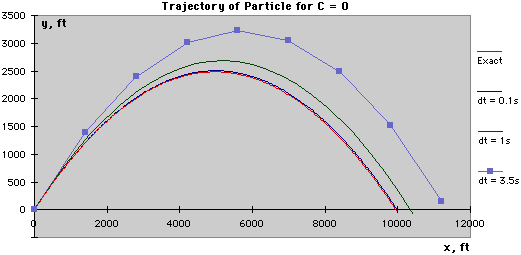Practice Homework and Test problems now available in the 'Eng Dynamics' mobile app
Includes over 400 problems with complete detailed solutions.
Available now at the Google Play Store and Apple App Store.